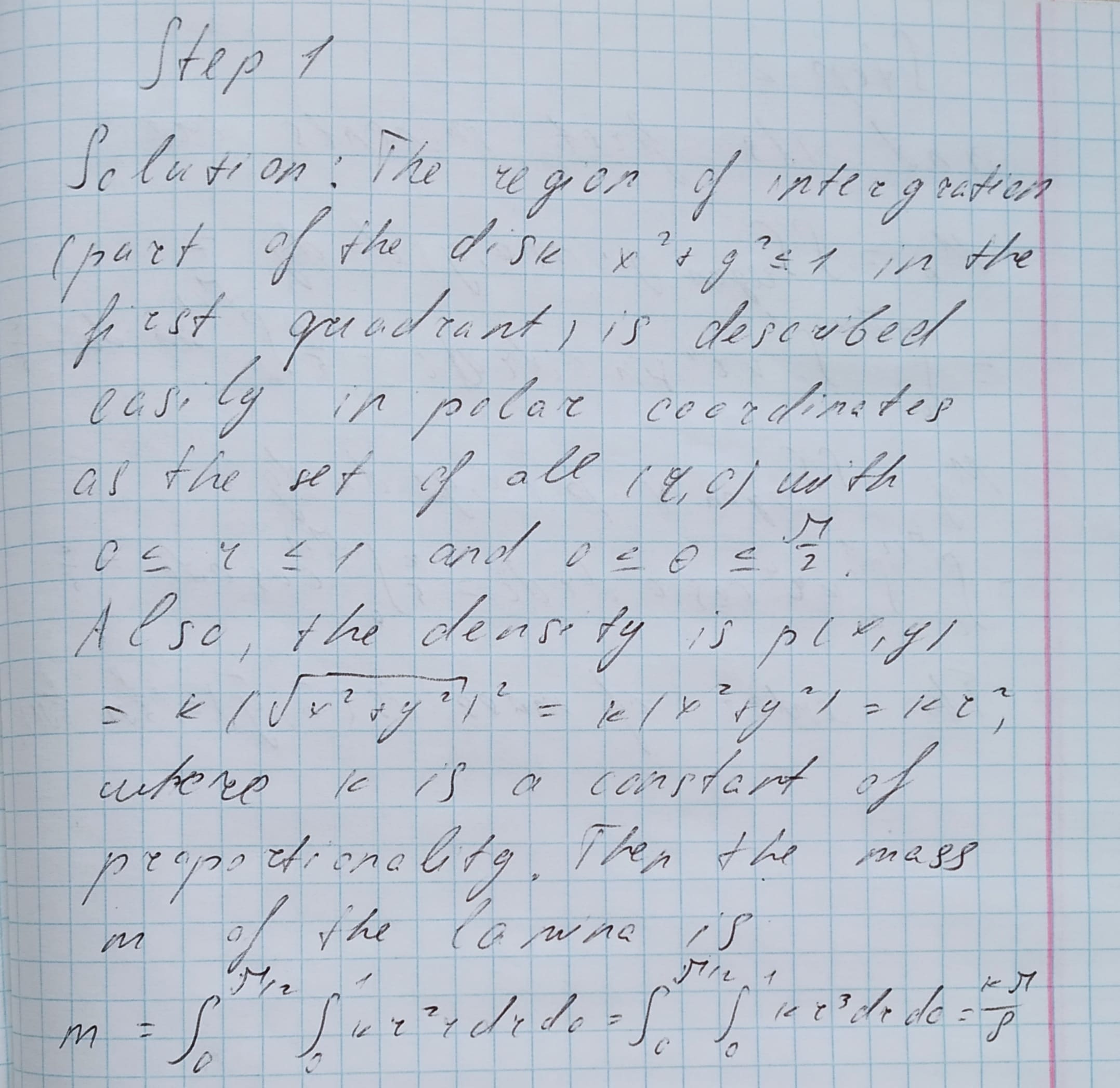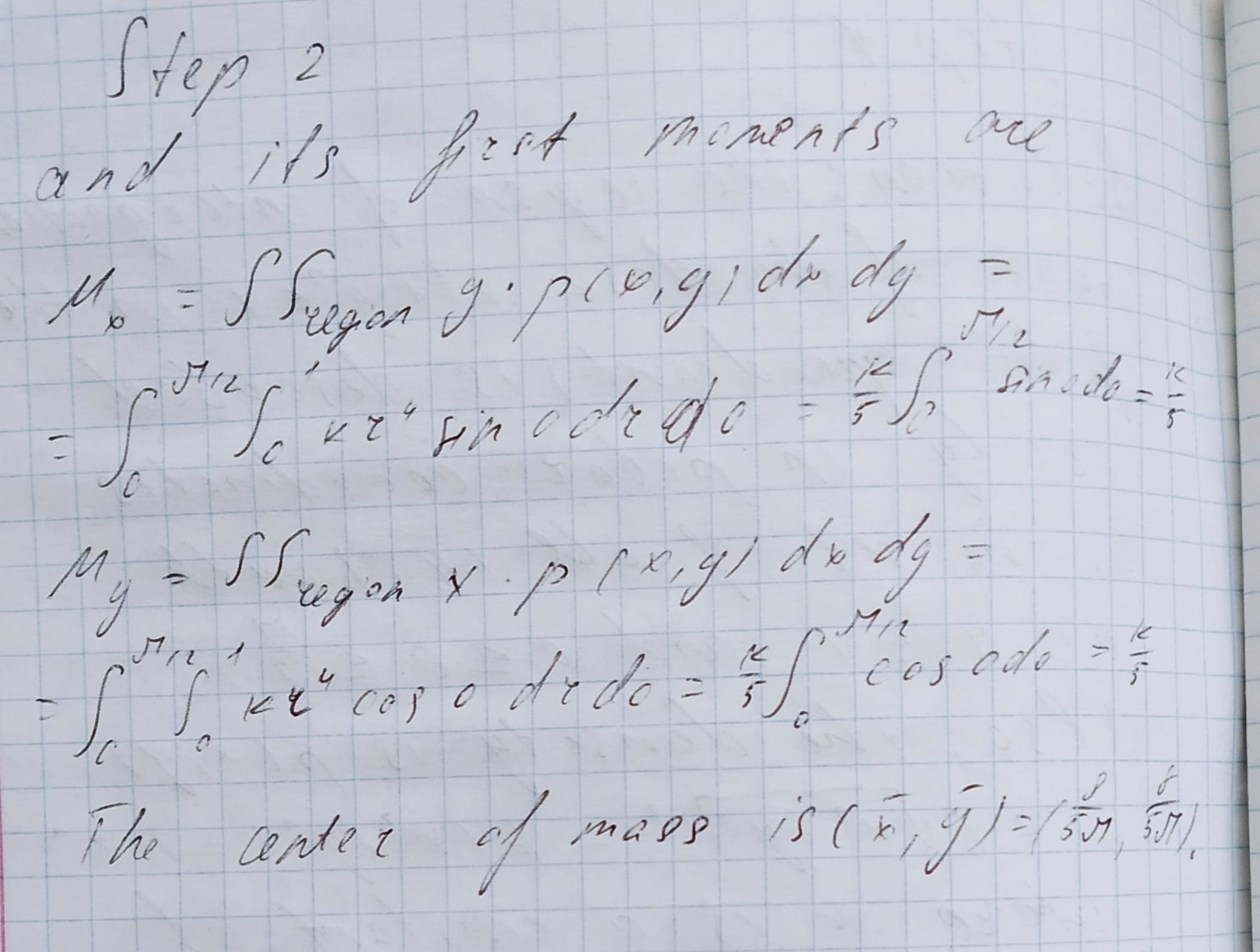# Applications using double integrals: A lamina occupies the part of the disk x^2 + y^2 <= 1 in the first quadrant. Use polar coordinates to find the center of mass of the lamina if the density at any point is proportional to the square of its distance from the origin.pedzenekO 2021-02-03 Answered
Applications using double integrals:
A lamina occupies the part of the disk ${x}^{2}+{y}^{2}\le 1$ in the first quadrant. Use polar coordinates to find the center of mass of the lamina if the density at any point is proportional to the square of its distance from the origin.
You can still ask an expert for help

• Questions are typically answered in as fast as 30 minutes

Solve your problem for the price of one coffee

• Math expert for every subject
• Pay only if we can solve itArnold Odonnell

...................................